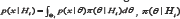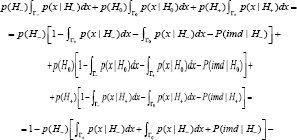info@biomedres.us   +1 (502) 904-2126   One Westbrook Corporate Center, Suite 300, Westchester, IL 60154, USA   Site Map

Impact Factor : 0.548

# On One Aspect of Constrained Bayesian Method for Testing Directional Hypotheses Volume 2 - Issue 5

KJ Kachiashvili*

• Georgian Technical University, Faculty of Informatics and Control Systems, Georgia

Received: February 23, 2018;   Published: March 06, 2018

*Corresponding author: KJ Kachiashvili, Georgian Technical University, Faculty of Informatics and Control Systems, st. Kostava, Tbilisi, Georgia

DOI: 10.26717/BJSTR.2018.02.000821

## Abstract

The paper discusses the application of constrained Bayesian method (CBM) of testing the directional hypotheses. It is proved that decision rule of CBM restricts the mixed directional false discovery rate (mdFDR) and total Type III error rate as well.

Keywords: Cbm; Hypotheses Testing; Mixed Directional False Discovery Rate; Type Iii Error Rate

## Introduction

Directional hypotheses testing problem arises in many biomedical applications . For parametrical models, the problem of testing directional hypotheses can be stated as Ho:θ = θ0 vs. H- :θ<θ1 or H+:θ>θ0, where θ is the parameter of the model, θ0 is known. A review of the works where the methods of testing the directional hypotheses are given can be found, for example, in [2,3]. Bayesian decision theoretical methodology for testing the directional hypotheses was developed and compared with the frequents method in . While a new approach to the statistical hypotheses testing, called Constrained Bayesian Methods (CBM), was developed and applied alongside to other types of hypotheses to the directional hypotheses in . One specific aspect of CBM for testing directional hypotheses, in particular, the fact that it restricts both the mixed directional false discovery rate (mdFDR) and total Type III error rate is proved below.

## Constrained Bayesian Method and Error Rates

Let's denote: г0 г_, г+ and are the regions of acceptance of the above-stated hypotheses 0 , H_ and H+ , respectively, and let us consider the following lossesWhere L1(Hi,Hj) and L2Hi,Hj) are the losses of incorrectly accepted and incorrectly rejected hypotheses. It is clear that the " 0-l" loss function is a private case of the step-wise loss (1). For loss functions (1), one of possible statement of CBM takes the form [3-5].Denotes the marginal density of x given Hi , i.e.is a priori density with supportis some real number determining the level of the averaged loss of incorrectly rejected hypothesis.By solving constrained optimization problem (5), (6), using the Lagrange multiplier method, we obtainWhere Lagrange multiplier λ is determined so that in  equality takes place. For optimality oftesting directional hypotheses, different concepts such as: mixed directional false discovery rate (mdFDR), directional false discovery rate (DFDR) and the Type III errors are offered in [1,6-10]. Let us consider mdFDR which is the exected proportion of falsely selecting H- or H+ . In our case, it has the following form### Theorem

When satisfying the conditionCBM with restriction level of  ensures a decision rule with mixed directional false discovery rate or with total Type III error rate less or equal to , i.e. with mdFDR = ERRTIII + ERRKIII ≤α .

Proof: Because of specificity of decision rules of CBM, alongside of hypotheses acceptance regions, the regions of impossibility of making a decision exist. Therefore, instead of the conditionWhere P(tmd| Hi) is the probability of impossibility of making a decision [3,11,12].

Taking into account , condition  can be rewritten as follows## Conclusion

One more property of the optimality of CBM when testing the directional hypotheses is shown. In particular, when testing the directional hypotheses, the optimal decision rule of CBM restricts both the mixed directional false discovery rate [mdFDR] and total Type III error rate.